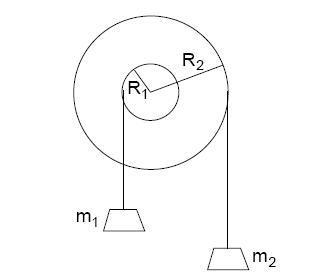# Angular Acceleration, 2 Pulleys on common axle

Note: This is similar to 2 previous questions I've seen here (https://www.physicsforums.com/showthread.php?t=261193"), and while this is my first post, I've lurked/found this site to really be helpful in getting me to be able to figure out how to solve problems I have with my physics homework.

## Homework Statement

Two pulley wheels, one of radius 0.3 m and the other of radius 0.82 m, are mounted rigidly on a common axle. The rotational intertia of the two pulleys, which are clamped together, is 3.3 kg·m². A mass of 44 kg mass is attached on the left and a mass of 37 kg mass on the right, as shown.Find the angular acceleration of the system. Take the clockwise direction to be positive. The acceleration of gravity is 9.8 m/s². Answer in units of rad/s².

ΣΤ = Iα

ΣΤ = Τ1 + Τ2

## The Attempt at a Solution

I found the torques for each mass & radius:

Τ1 = -(m1)g(R1)sin(90) --> (negative because going CCW)

Τ2 = (m2)g(R2)sin(90) --> (positive because going CW)

Therefore: ΣΤ = (m2)g(R2) - (m1)g(R1) = g(m2R2 - m1R1)

Which means: g(m2R2 - m1R1) = Iα

α = g(m2R2 - m1R1)/I

Checking the units, I should get rad/s²:

m/s²·(kg·m)/kg·m²

Which is: (m²·kg)/(s²·kg·m²)

So my units check out.

So, with the following variables (I'm hoping I got the left/right values correct):

• g = 9.8 m/s²
• m1 = 44 kg
• R1 = 0.3 m
• m2 = 37 kg
• R2 = 0.82 m
• I = 3.3 kg·m²

Plugging these in, I get:

α = [9.8]([0.82] - [0.3])/[3.3]

α = (9.8)(30.34 - 13.2)/3.3

α = (9.8)(17.14)/3.3

α = (167.972)/3.3

α = (167.972)/3.3

Which, apparently, is wrong. So I'm at a loss. What did I do wrong? Or rather, what would be a good hint for me to figure out where I went wrong?

(and yes, I know it seems strange for an α > g... that doesn't seem to logically follow for me)

Last edited by a moderator:

LowlyPion
Homework Helper
Welcome to PF.

Did you enter just 50.9?

Welcome to PF.

Did you enter just 50.9?

No, but the homework system my teacher uses is very annoying in needing an incredible number of sig figs. So I always have to just leave everything my calculator prints out to have success.

Update: Yes. It didn't help. I'm apparently missing something in my calculations.

Last edited: